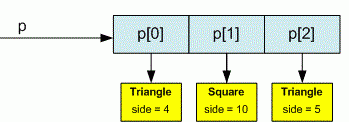Competitions

# Java Regular Polygon

Time limit 1 second
Memory limit 128 MiB
interface RegularPolygon {
int getNumSides();
double getSideLength();

static int totalSide(RegularPolygon[] rpArray) {
}

default double getPerimeter() {
}
default double getInteriorAngle() {
}
}

class EquilateralTriangle implements RegularPolygon {
private double sideLength;

public EquilateralTriangle(double sideLength) {
}

public int getNumSides() {
}

public double getSideLength() {
}

public String toString() {
}
}

class Square implements RegularPolygon {
private double sideLength;

public Square(double sideLength) {
}

public int getNumSides() {
}

public double getSideLength() {
}

public String toString() {
}
}

public class Main {
public static void main(String[] args) {
Scanner con = new Scanner(System.in);
int n = con.nextInt();
RegularPolygon[] rpArray = new RegularPolygon[n];
...
con.close();
}
}


Write an app with the following requirements:

• Create an interface called RegularPolygon with two abstract methods: getNumSides and getSideLength;

• Create a class EquilateralTriangle that implements the interface, has getNumSides return 3 and getSideLength return an instance variable that is set by the constructor;

• Write a class Square that implements the interface, has getNumSides return 4 and getSideLength return an instance variable that is set by the constructor;

• Add a static totalSides method to the interface, that given a RegularPolygon[], returns the sum of the number of sides of all the elements;

• getPerimeter (n * length, where n is the number of sides)

• getInteriorAngle ((n - 2 )π / n in radians)

## Input data

First line contains number n (n100) of geometric figures. Each next line contains the name of the figure (Triangle or Square) and its side length (double value).

## Output data

For each figure print its name and side, perimeter and value of interior angle like given in the output. In the last line print the number of sides in all figures.## Examples

Input example #1
3
Triangle 4
Square 10
Triangle 5

Output example #1
Triangle 4.0000
Perimeter: 12.0000
Interior angle: 1.0472
Square 10.0000
Perimeter: 40.0000
Interior angle: 1.5708
Triangle 5.0000
Perimeter: 15.0000
Interior angle: 1.0472
Total sides: 10

Author Michael Medvediev